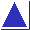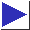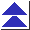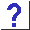# comp.lang.c FAQ list ·Question 3.14

Q: Why doesn't the code

```int a = 1000, b = 1000;
long int c = a * b;```
work?

A: Under C's integral promotion rules, the multiplication is carried out using int arithmetic, and the result may overflow or be truncated before being promoted and assigned to the long int left-hand side. Use an explicit cast on at least one of the operands to force long arithmetic:

```	long int c = (long int)a * b;
```
or perhaps
```	long int c = (long int)a * (long int)b;
```
(both forms are equivalent).

Notice that the expression (long int)(a * b) would not have the desired effect. An explicit cast of this form (i.e. applied to the result of the multiplication) is equivalent to the implicit conversion which would occur anyway when the value is assigned to the long int left-hand side, and like the implicit conversion, it happens too late, after the damage has been done.